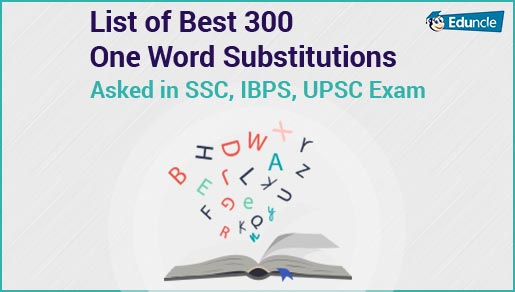Government Jobs FollowJuly 10, 2019 • 5:12 pm

# How to Solve Quadratic Equation Questions–Easy Tips, Tricks & MethodsThe Quadratic Equation is an important topic in the Quantitative Aptitude section. Questions from this topic are asked in almost every competitive exam.

So, candidates preparing for IBPS, SBI, SSC, RRB and other competitive exams must be looking for the easy and shortcut methods to solve Quadratic Equation questions.

To help those candidates, Eduncle has come up with this perfect guide for Quadratic Equation to solve the questions easily.

Below you can check the Quadratic Equation concept, shortcut methods and some examples to understand this topic deeply.

Start Preparation of Bank Exams 2019 with Free Study Stuff - Download Now!

## Quadratic Equation Definition – What is it?

Before you start solving the Quadratic Equation questions, you must know the definition of it. What actually is the Quadratic Equation.

So, a Quadratic Equation is an equation which contains at least one squared term. It can be defined in the form of ax2 + bx + c = 0. Where a, b and c are constants and ‘x’ is an unknown variable.

To clear the concept of Quadratic Equation, you must be familiar with all the terminologies that are used in this topic.

Below we have shared all such terminologies that are used in the Quadratic Equation questions.

A Quadratic Expression is something that can be simplified or factored. An expression cannot be solved for a variable but you can manipulate the terms that are given. Examples of a Quadratic Expression are:

x2 + 2x + 2

8x2 – 2x

4 – 5x – 10x2

6 – 12x2

A Quadratic Function can be represented in the form f(x) = ax2 + bx + c, where a, b & c are numbers with “a” not equal to zero. This function is given so that you can plot it on a graph. The graph of a quadratic equation is a Parabola.

So, these two are the most important terminologies that are used in the Quadratic Equation Questions. After getting aware of the terminologies it’s now time to check all the important formulas and basic concepts of Quadratic Equation.

Have a look.

Start Preparation of Bank Exams 2019-20 with Free Study Stuff & Video Lectures - Download Now!

## Quadratic Equation Formulas & Basic Concepts

Now here are some basic concepts and important Quadratic Equation Formulas that are used to solve the questions. You should learn all the formulas by heart as without them you will not be able to solve the questions.

The Quadratic Equation Questions can be solve using these two methods:

1. Standard Formula

2. Factorization Method

Let’s understand these Quadratic Equation Concepts deeply.

### 1. Standard Formula

This formula is used to solve the Quadratic Equations easily and quickly. If an equation is in the form ax² + bx + c = 0, then the solution will be x = -b ± √(b² -4ac)/2a.

You will get two values due to “+” and “-” sign. To understand this method better, we have given an example for it.

Let’s have a look.

Question: Solve for x: x2 + 4x – 8 = 0

Solution: Here in this equation, using the Standard formula, the values of a, b and c are 1, 4 and -8 respectively.

So, the solution will be:

x = -b ± √(b² -4ac)/2a

x = -(-4) ± √(4)2 – 4(1)(-8)/ 2(1)

x = 4 ±√16 + 32/2

x = 2 ± √48/2

x = 2 ± 2√12/2

x = 1 ± √12

Therefore, the two values of the equation are (1 + √12) and (1 – √12).

### 2. Factorization Method

In this method, you will have to find the factors of the given terms. This can only be solved when the given equation can be factorized. This method is also a quicker one to solve the Quadratic Equations.

Let’s study this method deeply with an example.

Question: Solve for x: x2 + 6x + 8 = 0

Solution: Here, in this equation we are using factorization method to solve the give equation. In this equation, b = 6 and c = 8.

You have to find the two numbers whose product will be 8 and sum will be 6. Now the factors of 8 are 1, 2, 4 and 8.

So, the two numbers will be 2 and 4.

Therefore,

x2 + 2x + 4x + 8 = 0

x(x + 2) + 4 (x + 2) = 0

So, the factors of the given equation are (x - 4) (x - 2).

Targeting Upcoming SSC Exams 2019-20? Get Free Study Materials and Previous Year Question Papers. Download Now.

These are the basic concepts, methods and formulas that are used to solve the Quadratic Equation Questions.

If you have any queries/doubts regarding this article, you can share them in the comments’ section below. We will get back to you as early as possible.

You Might Also Like